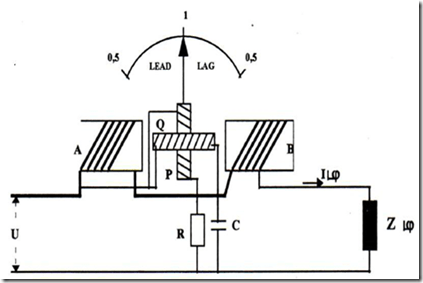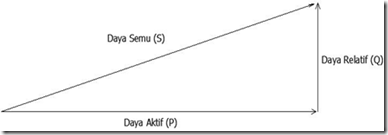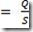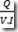Home > English > Configuration of Cos-Phi Meter Measurements

# Configuration of Cos-Phi Meter Measurements

Cos-Phi meter is a working power factor gauge for ac network that will measure a phase shift between voltage and current on an electrical load. In this case the cos-Phi meter gauge includes an electrodynamic type cross-coil instrument.

For the series of cos-Phi meters can be seen in the picture below:Figure 51. Cos-Phi Meter

Description of GB cos-Phi meter the number of loops P and Q is equal to the number where the coil P is serialized with resistance R and the coil Q is in series with resistance C, so that the current through the coil Q precedes the current through the 90 ° P coil of P. If the cos-Phi meter operates on a resistive load network the current flowing on the coil P will be in phase with the current flowing on the fixed coil AB so that the torque of the cross coil = 0.

Then the moving needle is calibrated cos-Phi = 1. But if the load is pure reactive (L or C). The current through the coil Q will be equivalent to the AB coil and the phase difference with the P coil is 90 degrees as a result of the cross (voltage) moving in such a way that the coil Q forms a new position perpendicular to the AB coil denoted by cos φ = 0. Next the needle will moving by φ degrees. If the load current I shifts by φ degrees to the load.

The direction of the clockwise pointer is indicated by (LAG) or precedes And counter-clockwise will be marked with (LEAD) or left behind Can be read on the scale board of the cos φ meter reading.

A. Type of power type of alternating current
For more details about the type of electrical power then below is described a triangle of power as follows.The angle between the active power vector and the apparent power is the phase shift angle and the ratio between the two vectors is called the power factor of the load (cos φ).

Sin φ=Where :
V = source voltage (Volt)
I = Flow current (ampere)
P = Active power (watts)
Q = Reactive power (VAR)
S = Pseudo Power (VA)

B. Improved power factor by calculating the capacitor value
An effort which can be made to minimize the phase shift between the current and the voltage (enlarge the power factor) is to increase the capacitor in parallel to the load because as we know that the current flowing at the capacity is opposite to the current flowing on the inductive load so that the current reactive would be smaller and if it could be expected to be zero. As it is known that reactive power:
QC = P (Tan φ 1 – Tan φ 2)
Where :
P = V. I. Cos φ
And
Q = V. I (Tan φ 1)"Tasikmalaya, Indonesia"